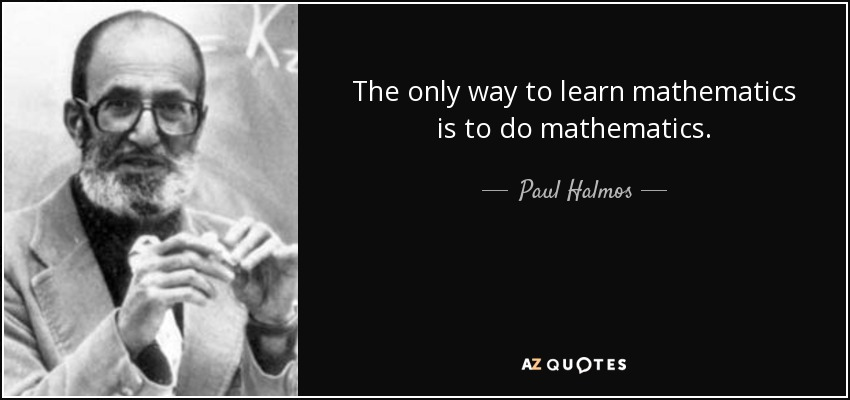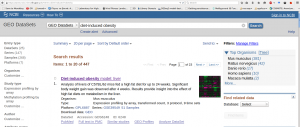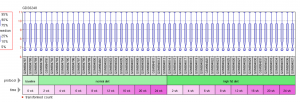# Installing R

R is a free and open source programming language. You can find download instructions here:

https://www.r-project.org/

• Try to install it in your personal computer

Note: If you are using Linux (some debian flavor) then installing R is really simple:

`sudo apt-get install r-base r-base-core`

# Using R for the course

• If R is installed in the University Computers, you can use R there.
• You can also login to:
139.91.162.50:8787

# Statistics for BioinformaticsSo, just read and try…. it’s the best way to learn applying statistics in bioinformatics problems.

# Why Statistics in Biology

• Many students go to Biology to avoid anything related to math.
• Why do you have now to learn statistics and apply them to biological data?

# Statistics is the Science of Learning from Data

You use a small collection (a sample) to learn something for the whole (the population).

Sample (Wikipedia):
In statistics and quantitative research methodology, a data sample is a set of data collected and/or selected from a statistical population by a defined procedure.A sample can be random: The chance of sampling an object does not depend on the properties of the object.

• The sample is taken from a population.
• The population can be assumed something large, perhaps infinite, and unknown.
• We are interested in understanding parameters from the population.
• We study the sample in order to understand the population.You can click on the dataset. Then, go to the bottom of the page:
Experiment design and value distribution

Click on the experimental design. You will see something similar to that:

#This represents the design of the study. Let’s try to understand it:

• 3 protocols: baseline, normal diet, high fat dies
• several time-points (after birth)

Notes

• When we compare the diets, the baseline can be neglected (there is no diet there)
• Expression values of the same at different samples are different due to:
• different time-point
• different treatment (diet)
• stochasticity

# Understanding our data

## Descriptive quantities for the gene expression values

In R, it is very easy to calculate descriptive statistics:

• mean, variance, standard deviation, percentiles

It is also very easy to summarize the data using:

• boxplots, histograms, densities

Visualization

With R it is easy to visualize the data:

• Principal Component Analysis
• Multidimensional Scaling
• Heatmaps

# Hypothesis testing

Now, it’s time to perform some hypothesis testing:

For example,

• geneA is higher in high-fat diet mice, than normal fat diet mice

In general, to perform a hypothesis testing we need:

• A hypothesis to test, that is formed prior to seeing the data
• Data
• A statistic
• A theoretical distribution of the statistic under the null hypothesis
• A significance threshold